{{announcement.body}}
{{announcement.title}}

# Classification From Scratch, Part 8 of 8: Linear Discrimination

DZone 's Guide to

# Classification From Scratch, Part 8 of 8: Linear Discrimination

### We continue our article series on using R for creating regression and classification models from scratch by looking at Bayes/Naive Classifiers and Linear Regression.

· Big Data Zone ·
Free Resource

Comment (0)

Save
{{ articles.views | formatCount}} Views

This is the eight post of our series on classification from scratch. The latest one was on SVM, and today, I want to get back to some very old stuff and also a linear separation of the space, using Fisher’s linear discriminant analysis.

## Bayes (Naive) Classifier

Consider the following naive classification rule: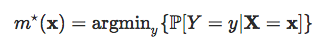or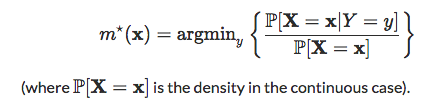In the case where y takes two values, that will be standard {0,1} here, one can rewrite the later as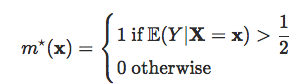and the set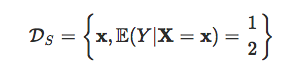is called the decision boundary.

Assume that

XY=0∼N(μ0,Σ)

and

XY=1∼N(μ1,Σ

then explicit expressions can be derived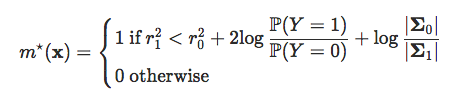where ry2 is the Manalahobis distance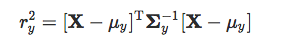Let δbe defined as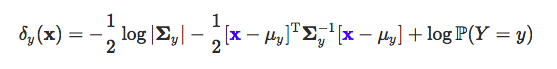the decision boundary of this classifier is

{x such that δ0(x)=δ1(x)}

which is quadratic in x. This is the quadratic discriminant analysis. This can be visualized as below.The decision boundary is here:But that can’t be the linear discriminant analysis, right? I mean, the frontier is not linear… Actually, in Fisher’s seminal paper, it was assumed that Σ0=Σ1.

In that case, actually,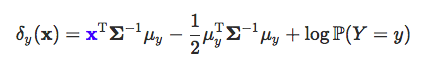and the decision frontier is now linear in x. This is the linear discriminant analysis. This can be visualized as below:Here the two samples have the same variance matrix and the frontier is:## Link With the Logistic Regression

Assume as previously that

XY=0∼N(μ0,Σ)

and

\XY=1∼N(μ1,Σ

then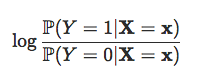is equal to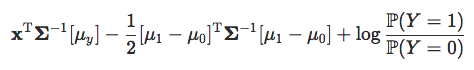which is linear in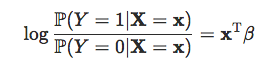Hence, when each group has Gaussian distributions with an identical variance matrix, then LDA and the logistic regression lead to the same classification rule.

Observe furthermore that the slope is proportional to Σ−1[μ1−μ0], as stated in Fisher’s article. But to obtain such a relationship, he observes that the ratio of between and within variances (in the two groups) was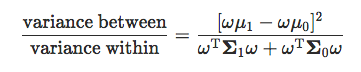which is maximal when ω is proportional to Σ−1>[μ1−μ0], when Σ0=Σ1.

## Homebrew Linear Discriminant Analysis

To compute vector ω

m0 = apply(myocarde[myocarde$PRONO=="0",1:7],2,mean) m1 = apply(myocarde[myocarde$PRONO=="1",1:7],2,mean)
Sigma = var(myocarde[,1:7])
omega = solve(Sigma)%*%(m1-m0)
omega
[,1]
FRCAR -0.012909708542
INCAR  1.088582058796
INSYS -0.019390084344
PRDIA -0.025817110020
PAPUL  0.020441287970
PVENT -0.038298291091
REPUL -0.001371677757

For the constant – in the equation ω^x+b=0 – if we have equiprobable probabilities, use

b = (t(m1)%*%solve(Sigma)%*%m1-t(m0)%*%solve(Sigma)%*%m0)/2

## Application (on the Small Dataset)

In order to visualize what’s going on, consider the small dataset, with only two covariates:

x = c(.4,.55,.65,.9,.1,.35,.5,.15,.2,.85)
y = c(.85,.95,.8,.87,.5,.55,.5,.2,.1,.3)
z = c(1,1,1,1,1,0,0,1,0,0)
df = data.frame(x1=x,x2=y,y=as.factor(z))
m0 = apply(df[df$y=="0",1:2],2,mean) m1 = apply(df[df$y=="1",1:2],2,mean)
Sigma = var(df[,1:2])
omega = solve(Sigma)%*%(m1-m0)
omega
[,1]
x1 -2.640613174
x2  4.858705676Using R regular functions, we get:

library(MASS)
fit_lda = lda(y ~x1+x2 , data=df)
fit_lda

Coefficients of linear discriminants:
LD1
x1 -2.588389554
x2  4.762614663

which is the same coefficient as the one we got with our own code. For the constant, use:

b = (t(m1)%*%solve(Sigma)%*%m1-t(m0)%*%solve(Sigma)%*%m0)/2

If we plot it, we get the red straight line

plot(df$x1,df$x2,pch=c(1,19)[1+(df$y=="1")]) abline(a=b/omega,b=-omega/omega,col="red")As we can see (with the blue points), our red line intersects the middle of the segment of the two barycenters: points(m0["x1"],m0["x2"],pch=4) points(m1["x1"],m1["x2"],pch=4) segments(m0["x1"],m0["x2"],m1["x1"],m1["x2"],col="blue") points(.5*m0["x1"]+.5*m1["x1"],.5*m0["x2"]+.5*m1["x2"],col="blue",pch=19) Of course, we can also use an R function: predlda = function(x,y) predict(fit_lda, data.frame(x1=x,x2=y))$class==1
vv=outer(vu,vu,predlda)
contour(vu,vu,vv,add=TRUE,lwd=2,levels = .5)One can also consider the quadratic discriminant analysis since it might be difficult to argue that Σ0=Σ1.

fit_qda = qda(y ~x1+x2 , data=df)

The separation curve is here:

plot(df$x1,df$x2,pch=19,
col=c("blue","red")[1+(df$y=="1")]) predqda=function(x,y) predict(fit_qda, data.frame(x1=x,x2=y))$class==1
vv=outer(vu,vu,predlda)
contour(vu,vu,vv,add=TRUE,lwd=2,levels = .5)Topics:
bayes classifier, big data, data classification, r

Comment (0)

Save
{{ articles.views | formatCount}} Views

Published at DZone with permission of Arthur Charpentier , DZone MVB. See the original article here.

Opinions expressed by DZone contributors are their own.